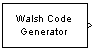# Walsh Code Generator

Generate Walsh code from orthogonal set of codes

## Library

Sequence Generators sublibrary of Comm Sources## Description

Walsh codes are defined as a set of N codes, denoted Wj, for j = 0, 1, ... , N - 1, which have the following properties:

• Wj takes on the values +1 and -1.

• Wj = 1 for all j.

• Wj has exactly j zero crossings, for j = 0, 1, ... , N - 1.

• ${W}_{j}{W}_{k}^{T}=\left\{\begin{array}{cc}0& j\ne k\\ N& j=k\end{array}$

• Each code Wj is either even or odd with respect to its midpoint.

Walsh codes are defined using a Hadamard matrix of order N. The Walsh Code Generator block outputs a row of the Hadamard matrix specified by the Walsh code index, which must be an integer in the range [0, ..., N - 1]. If you set Walsh code index equal to an integer j, the output code has exactly j zero crossings, for j = 0, 1, ... , N - 1.

Note, however, that the indexing in the Walsh Code Generator block is different than the indexing in the Hadamard Code Generator block. If you set the Walsh code index in the Walsh Code Generator block and the Code index parameter in the Hadamard Code Generator block, the two blocks output different codes.

## Parameters

Code length

Integer scalar that is a power of 2 specifying the length of the output code.

Code index

Integer scalar in the range [0, 1, ... , N - 1], where N is the Code length, specifying the number of zero crossings in the output code.

Sample time

The time between each sample of the output signal. Specify as a nonnegative real scalar.

Samples per frame

The number of samples in one column of the output signal. If Samples per frame is greater than the Code length, the code is cyclically repeated. Specify as a positive integer scalar.

### Note

The time between output updates is equal to the product of Samples per frame and Sample time. For example, if Sample time and Samples per frame equal one, the block outputs a sample every second. If Samples per frame is increased to 10, then a 10-by-1 vector is output every 10 seconds. This ensures that the equivalent output rate is not dependent on the Samples per frame parameter.

Output data type

The output type of the block can be specified as an `int8` or `double`. By default, the block sets this to `double`.